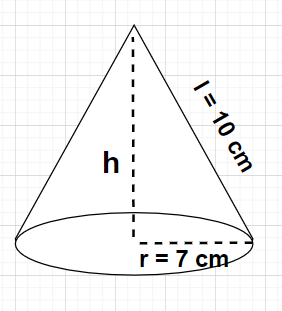Courses
Courses for Kids
Free study material
Offline Centres
MoreLast updated date: 05th Dec 2023
Total views: 384.3k
Views today: 6.84k

# The radius and slant height of a cone are 7 cm and 10 cm respectively. Find its curved surface area. ($\pi = \dfrac{{22}}{7}$ )Verified
384.3k+ views
Hint: Here, we will be proceeding by drawing a diagram using the given data (radius and slant height of the cone) and determine the curved surface area of this cone with the help of formula for the curved surface area of the cone i.e., ${\text{C}}{\text{.S}}{\text{.A}} = \pi rl$.${\text{C}}{\text{.S}}{\text{.A}} = \pi rl$
${\text{C}}{\text{.S}}{\text{.A}} = \pi rl = \dfrac{{22}}{7} \times 7 \times 10 = 220{\text{ c}}{{\text{m}}^2}$
Note: In any cone with radius r and height h, the slant height h is given by $l = \sqrt {{r^2} + {h^2}}$. For calculation of curved surface area of the cone (${\text{C}}{\text{.S}}{\text{.A}} = \pi rl$), radius and slant height of the cone is required. But if instead of slant height l, height of the cone h is given then we will be using formula $l = \sqrt {{r^2} + {h^2}}$ to find the value of slant height first.